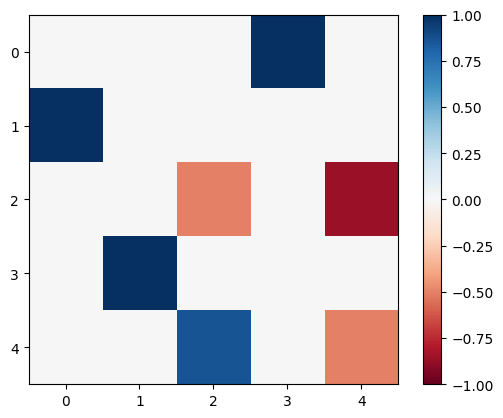Change of Basis

In the release 0.2.2, the euler angle convention changed from the standard ZYZ to YXY. This amounts to a change of basis for e3nn.

This change of basis means that the real spherical harmonics have been rotated from the “standard” real spherical harmonics (see this table of standard real spherical harmonics from Wikipedia). If your network has outputs of L=0 only, this has no effect. If your network has outputs of L=1, the components are now ordered x,y,z as opposed to the “standard” y,z,x.

If, however, your network has outputs of L=2 or greater, things are a little trickier. In this case there is no simple permutation of spherical harmonic indices that will get you back to the standard real spherical harmonics.

In this case you have two options (1) apply the change of basis to your inputs or (2) apply the change of basis to your outputs.

1. If the only inputs you have are scalars and positions, you can just permute the indices of your coordinates. You just need to permute from y,z,x to x,y,z. If you choose this method, be careful. You must keep the permuted coordinates for all subsequent analysis calculations.

2. If you want to apply the change of basis more generally, for higher L, you can grab the appropriate rotation matrices, like this example for L=2:

import torch
from e3nn import o3
import matplotlib.pyplot as plt

change_of_coord = torch.tensor([
# this specifies the change of basis yzx -> xyz
[0., 0., 1.],
[1., 0., 0.],
[0., 1., 0.]
])

D = o3.Irrep(2, 1).D_from_matrix(change_of_coord)

plt.imshow(D, cmap="RdBu", vmin=-1, vmax=1)
plt.colorbar();Of course, you can apply the rotation method to either the inputs or the outputs – you will get the same result.